# Multiplication Methods

This times table blog gives you the teaching progression for learning multiplication in primary schools. It takes you through the different multiplication methods that are taught and challenges you to answer multiplication questions. Let us know your answers in the comments section!

## What are times tables?

Times tables are a list of multiples of a number. For example, 2, 4, 6, 8, and 10 are all multiples of 2. They are all part of the two times tables.

## What vocabulary is associated with times tables?

The vocabulary used to mean ‘times’ includes:

• multiply
• lots of
• groups of
• sets of?
• multiplied by
• array
• product
• times table

## What are times table facts?

A times table fact is the answer to a multiplication number sentence. Each fact has at least three other facts related to it.  When you learn 2 x 4 = 8 you also know 4 x 2 = 8, 8 ÷ 4 = 2, 8 ÷ 2 = 4.

## How do I solve a multiplication question?

Multiplication questions can be solved in lots of different ways, including using concrete objects, pictorial representations, repeated addition, knowledge of number facts and methods like long multiplication.

### Concrete Objects

To solve a multiplication problem with objects you need to select objects to represent the number sentence.

This picture shows four groups of 3 or 3 + 3 + 3 + 3 or 4 x 3. Can you work out the answer?What is the answer to 4 lots of 3?

You can use the photos of objects or you can get your own objects and arrange them into groups before you count them.

### Pictorial Representation

To solve a multiplication problem using pictures you need to draw objects to represent the number sentence.

This picture shows five groups of 4  or  4 + 4 + 4 + 4 + 4  or  5 x 4. Can you work out the answer?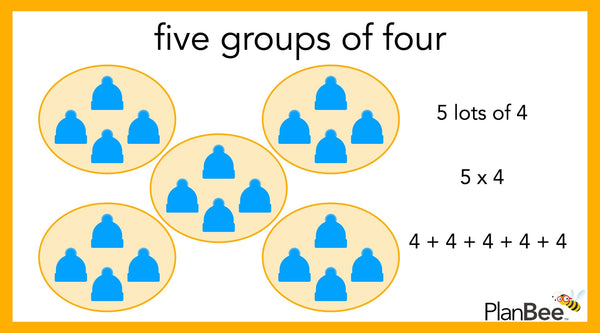What is the answer to 5 groups of 4?

You can use the photo or you can draw your own pictures to help you solve this number sentence.

### Repeated Addition Multiplication

To solve a multiplication problem using repeated addition you can either add the same number again and again by counting in ones or you can count in multiples.

Eight groups of two is 8 x 2  or  2 + 2 + 2 + 2 + 2 + 2 + 2 + 2. Can you work out the answer?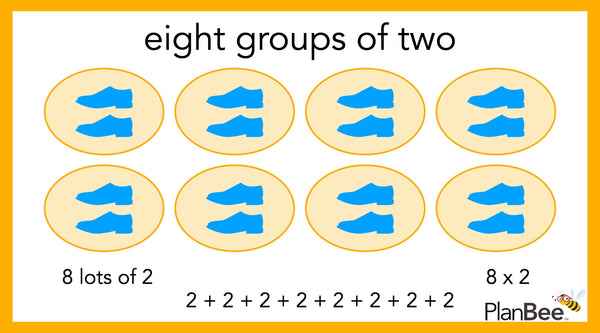What is the answer to 8 groups of 2?

If you want to know more about using concrete objects, pictorial representations, or repeated addition to solve multiplication problems have a look at our Year 1 Maths schemes ‘Let’s count in multiples’, ‘Let’s find the total by grouping’ and ‘Let’s share objects equally'.

These ready-to-teach multiplication and division lesson packs all about contain detailed planning, informative teaching slides, and engaging worksheets all designed to cover Year 1 multiplication and division Maths National Curriculum objectives.

### Number Facts Multiplication

To solve a multiplication problem using number facts you need to use the number facts you know. This might involve using your knowledge of times tables, doubles, or rounding.

To solve nine multiplied by eight you might use your knowledge of doubling or rounding and the ten times table.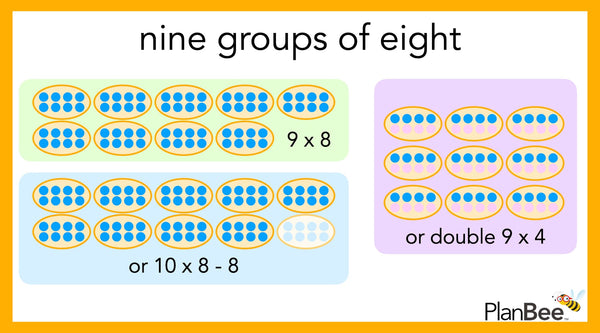What is the answer to 9 groups of 8?

9 x 8 = ?

Nine multiplied by eight is the same as ten multiplied by eight minus eight.

10 x 8 = 80  so  9 x 8 = 10 x 8 - 8 = 80 - 8 = 72

Nine multiplied by eight is the same as double nine multiplied by four.

9 x 4 = 36 double that to get 9 x 8 = 72

Which method would you use to work out the answer?

If you want to know more about using number facts and fact families to solve problems have a look at our Year 2 Maths scheme ‘Let’s multiply and divide’.

This ready-to-teach lesson pack on solving multiplication and division problems, contains detailed planning, informative teaching slides, and engaging worksheets all designed to cover Year 2 Maths National Curriculum objectives.

### Partitioning Multiplication

Partitioning numbers is a helpful way to solve multiplication problems involving numbers larger than one-digit numbers.

To solve seventy-three multiplied by six using partitioning you might separate seventy-three into tens and ones. Then you multiply seventy (or seven tens) by six and three by six. Then you add the two answers together.

73 x 6 = ?

70 x 6 = 420

3 x 6 =  18

420 + 18 = 400 + 20 + 0 + 10 + 8 = 438

73 x 6 = 438Solve 73 x 6 using partitioning

If you need help becoming confident with place value download these free Place Value Cards and this free Place Value Game

Now let’s try solving a multiplication problem with a 3-digit number using partitioning.

To solve one hundred and twenty-four multiplied by four using partitioning you might separate one hundred and twenty-four into hundreds, tens, and ones. Then you multiply one hundred by four, twenty (or two tens) by four, and four by four. Then you add the three answers together.

124 x 4 = ?

100 x 4 = 400

20 x 4 = 80

4 x 4 =  16

400 + 80 + 16 = 400 + 80 + 10 + 6 = 496

124 x 4 = 496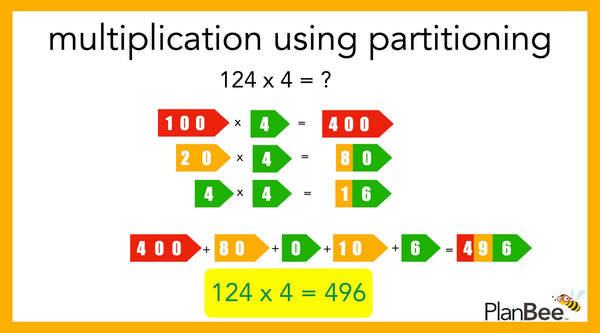Solve 124 x 4 using partitioning

If you want to know more about using partitioning to solve problems have a look at our Year 3 Maths scheme ‘Multiplying and Dividing’.

This ready-to-teach lesson pack introduces the concept of multiplying 2-digit numbers by a 1-digit number using partitioning. It contains detailed planning, informative teaching slides, and engaging worksheets all designed to cover Year 3 Maths National Curriculum objectives.

### The Grid Method Multiplication

The grid method is a helpful way to solve multiplication problems involving numbers larger than 1-digit numbers. This method builds on your knowledge of partitioning.

Can you solve 53 x 8 using the grid method? Use the example below to help you.Solve 66 x 8 using the grid method

If you want to know more about using the grid method to solve problems have a look at lesson 5 of our Year 3 Maths scheme ‘Using Times Tables’.

This ready-to-teach lesson pack introduces the grid method to solve multiplication problems. It contains detailed planning, informative teaching slides, and engaging worksheets all designed to cover Year 3 Maths National Curriculum objectives.

### The Expanded Method

Partitioning numbers is a helpful way to solve multiplication problems involving numbers larger than 1-digit numbers.

Can you solve 73 x 9 using the expanded method? Use the example below to help you.Solve 67 x 8 using the expanded method

If you want to know more about using partitioning to solve problems have a look at lesson 2 of our Year 4 Maths scheme ‘Multiplication and Division Methods’.

This ready-to-teach lesson pack introduces using the expanded method (and lots of other methods!) to solve multiplication problems. It contains detailed planning, informative teaching slides, and engaging worksheets all designed to cover Year 4 Maths National Curriculum objectives.

### Informal Partitioning Multiplication

Informal partitioning is a way to solve multiplication problems by partitioning. This version of partitioning requires a good understanding of place value and partitioning because fewer steps are recorded.

Can you solve 39 x 6 using the informal partitioning method? Use the example below to help you.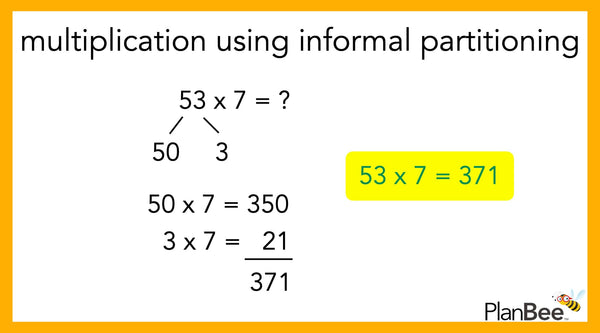Solve 53 x 7 using informal partitioning

If you want to know more about using informal partitioning to solve problems have a look at lesson 3 and lesson 4 our Year 4 Maths scheme ‘Seeing Doubles’.

This ready-to-teach lesson pack introduces the concept of multiplying 2-digit numbers by a 1-digit number using informal partitioning. It contains detailed planning, informative teaching slides, and engaging worksheets all designed to cover Year 4 Maths National Curriculum objectives.

### Short Multiplication

We can use short multiplication to multiply any number by a one-digit number. Working from right to left, the ONES, TENS, HUNDREDS, and so on are multiplied. If the product of sum is more than 9 we carry the number.

Can you solve 95 x 6 using short multiplication? Use the example below to help you.Solve 73 x 6 using short multiplication

If you want to know more about using short multiplication to solve problems have a look at lesson 4 of our Year 4 Maths scheme ‘Multiplying Doubles and Digits’.

This ready-to-teach lesson pack introduces using short multiplication to solve multiplication problems. It contains detailed planning, informative teaching slides, and engaging worksheets all designed to cover Year 4 Maths National Curriculum objectives.

### Long Multiplication Method

Long multiplication or formal multiplication is a helpful way to solve multiplication problems when both numbers are larger than 1-digit numbers. Long multiplication is very similar to short multiplication except that you have to complete more than one set of multiplications. Just as before, we start with the ones, then the tens, and so on, and we carry the numbers if the product is more than 9.

Can you solve 395 x 5 using long multiplication? Use the example below to help you.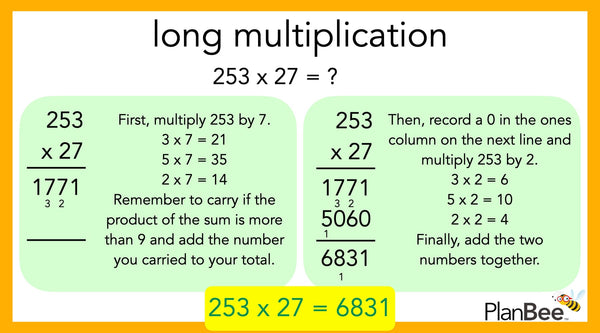Solve 253 x 27 using long multiplication

If you want to know more about using long multiplication to solve problems have a look at lesson 4 of our Year 5 Maths scheme ‘Formal Multiplication’.

This ready-to-teach lesson pack introduces using long multiplication to solve multiplication problems. It contains detailed planning, informative teaching slides, and engaging worksheets all designed to cover Year 5 Maths National Curriculum objectives.

## Download these FREE multiplication resources and times tables games

These free multiplication resources can help you become more confident with your times tables.

These Times Table Grids and Multiplication Square can help you learn and practice multiplication tables. The free pack includes a completed multiplication grid, a blank multiplication grid, a mixed-up multiplication grid, and a multiplication grid with missing numbers.

These Times Table Cubes can help you learn and practice the 2 times table to the 12 times table.

This Times Table Booklet is a fun way to check and develop your knowledge of multiplication facts up to 12 x 12.

This Times Table Chart is a great reference to have when you are learning multiplication facts up to 12 x 12.

This Multiplication Wheel resource is a fun way for your class to practice their times table knowledge, as well as self-check their answers.

This Multiplication Loop Game is a great way to practice multiplication skills during mental oral starters.

This Multiplication Make a Square Game is a really fun way to practice multiplication skills.

## Want ready-to-teach Maths lesson packs?!

We’ve got loads of downloadable Maths lesson packs. Each pack comes with lesson plans, slide show presentations and worksheets. View our full collection of Multiplication and Division lesson packs and learn how to get the most out of our PDF resources

### Related Posts##### Anglo-Saxon KS2 Facts
Where did the Anglo-Saxons come from? What did the Anglo-Saxons eat? What did they wear? Find out some fascinating facts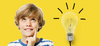##### How to make children more energy aware
With the current energy crisis in full swing, and costs rising at an alarming rate for schools who are already strugglin